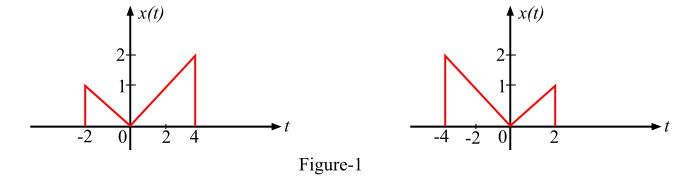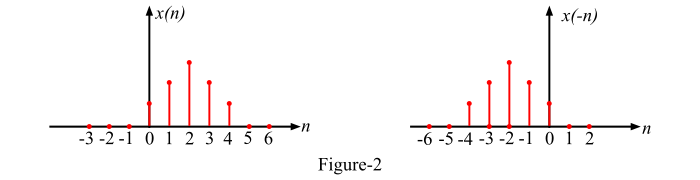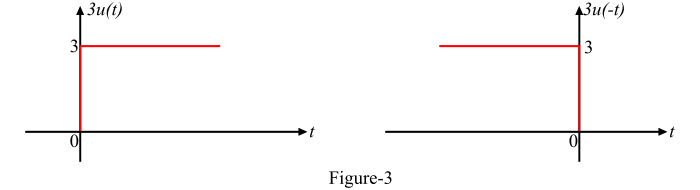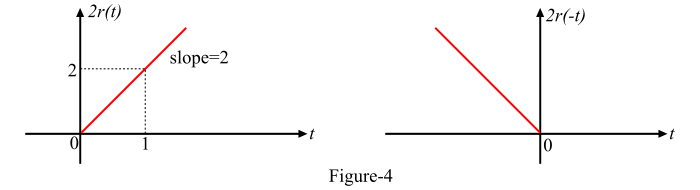# What is the Time Reversal Operation on Signals?

## What is Time Reversal of a Signal?

The time reversal of a signal is folding of the signal about the time origin (or t = 0). The time reversal or folding of a signal is also called as the reflection of the signal about the time origin (or t = 0). Time reversal of a signal is a useful operation on signals in convolution.

## Time Reversal of a Continuous-Time Signal

The time reversal of a continuous time signal x(t) is the rotation of the signal by 180° about the vertical axis. Mathematically, for the continuous time signal x(t), the time reversal is given as,

𝑦(𝑡) = 𝑥(−𝑡)

An arbitrary continuous-time signal x(t) and its time reversal x(-t) are shown in Figure-1.## Time Reversal of a Discrete-Time Sequence

For a discrete time sequence x(n), the time reversal is given by,

𝑦(𝑛) = 𝑥(−𝑛)

An arbitrary discrete-time signal x(n) and its time reversal x(-n) are shown in Figure-2.## Numerical Example

Sketch the following signals −

• 𝑥(𝑡) = 3𝑢(−𝑡)
• 𝑥(𝑡) = 2𝑟(−𝑡)

### Solution

• Given signal is,

𝑥(𝑡) = 3𝑢(−𝑡)

The given signal [3𝑢(−𝑡)] can be obtained by first drawing the step signal 3𝑢(𝑡) and then time reversing the signal 3𝑢(𝑡) about the time origin (i.e., t = 0) to obtain the signal 3𝑢(−𝑡) as shown in Figure-3.• Given signal is,

𝑥(𝑡) = 2𝑟(−𝑡)

The given signal [2𝑟(−𝑡)] can be obtained by first drawing the ramp signal 2𝑟(𝑡) as shown in Figure-4. Then time reversing or folding the signal 2𝑟(𝑡) about the time origin (i.e., t = 0) to obtain [2𝑟(−𝑡)] as shown in Figure-4.